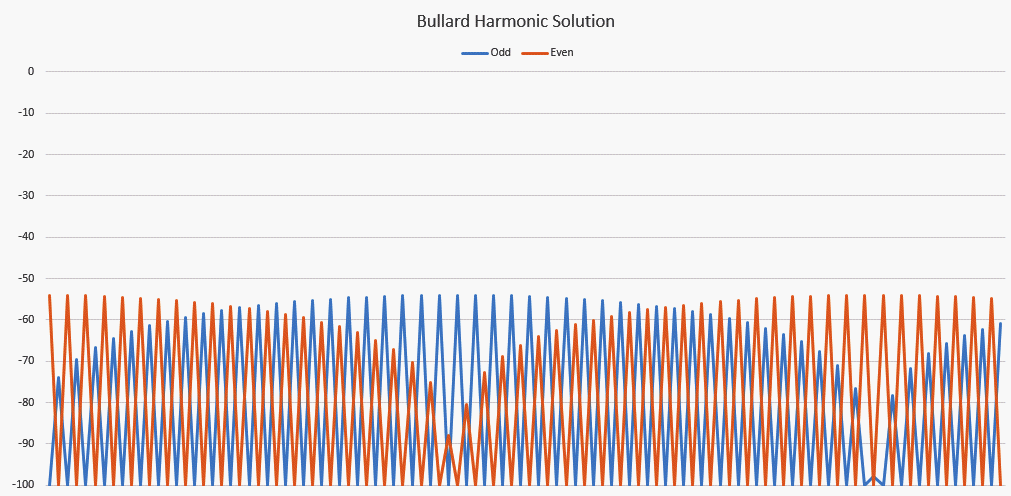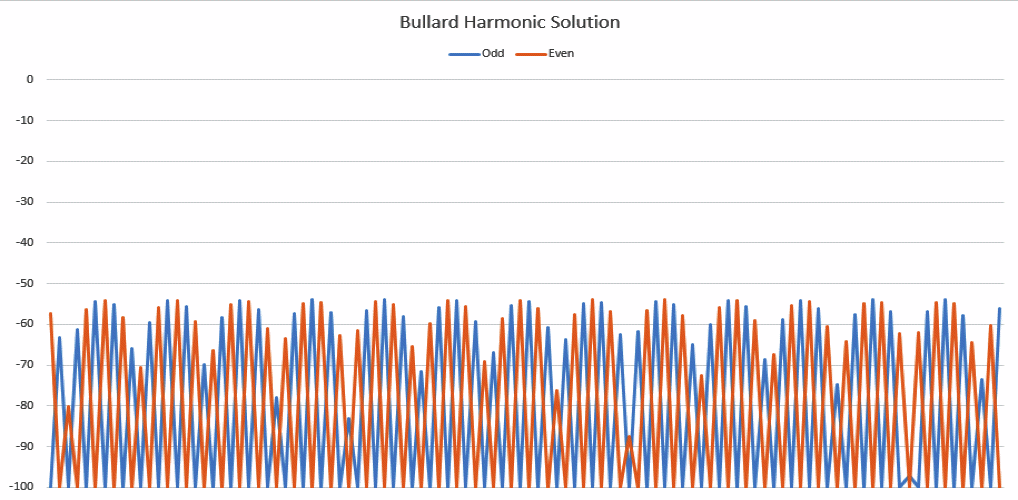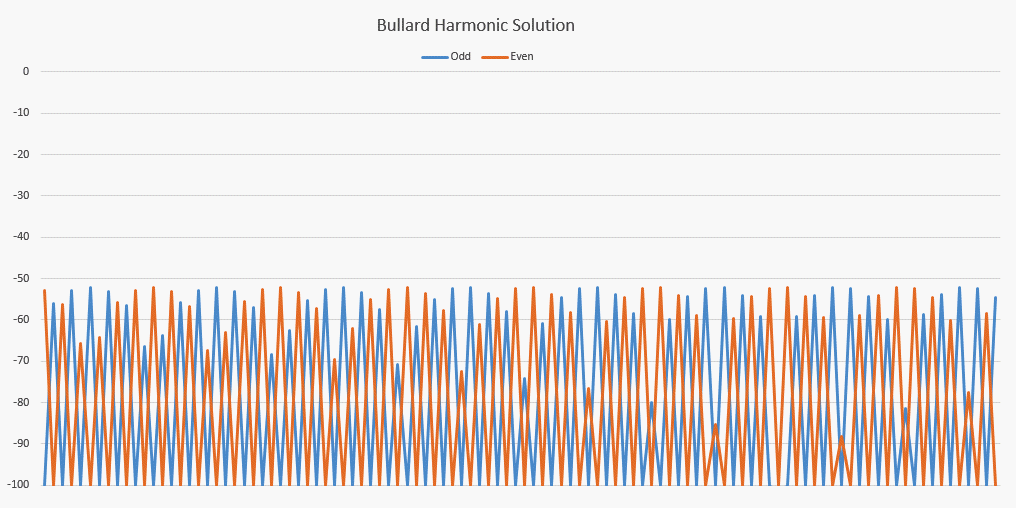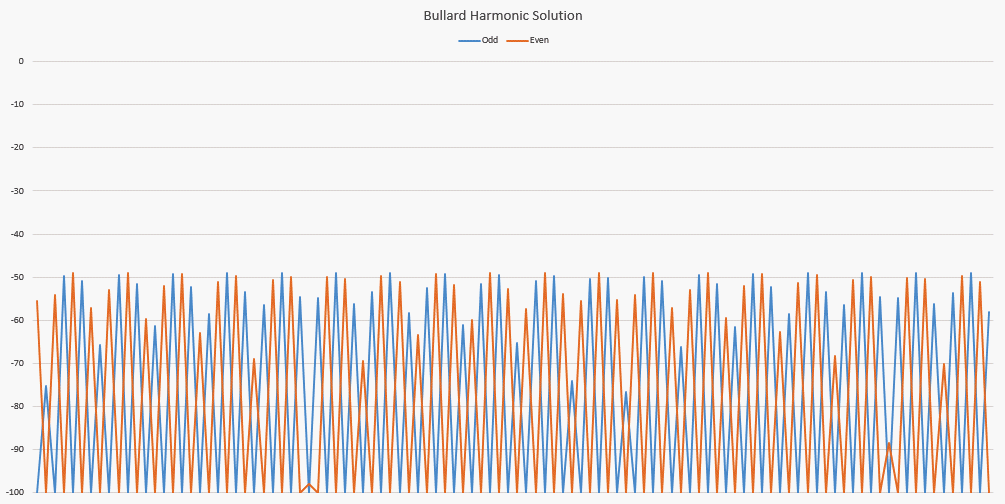Bullard Harmonic Solution vs a real spectrum for a specific distortion

# The Validity Of The Bullard Harmonic Solution

Published on November 12, 2019

Once I figured out the right formula for predicting the harmonics of a square and rectangular wave, I thought I could find the right formula for predicting the harmonics of a triangle/ramp wave. And once I figured that out, I was half way there to coming up with a formula for predicting the harmonics for a distorted sine wave. That became the Bullard Harmonic Solution, which I am not going to give you here. But you can get it for as little as \$7 so don't fret too much. My point here is to show that it very accurately predicts the harmonics of a distortion at any angle in a sine wave.

So here I am going to show you a few animated GIFs that include both the spectrum of a distorted sine wave and the Bullard Harmonic Solution prediction of the same distortion. There are two things to keep in mind when looking at these plots. 1) The Bullard Harmonic Solution does not predict the amplitude of the fundamental or the DC offset. The BHS (for short) only predicts harmonics, not the fundamental or DC offset, and 2) the BHS only predicts the harmonics created by a single distortion at a single angle. In the books Distortion and Harmonics I explain that a peak clipping looks slightly different than the BHS prediction because the actual width of the distortion is quite wide, causing the low frequencies to be boosted in amplitude. This happens in any distortion that is wider than just a single point. In the books I suggest some ideas for coming up with a way to predict the harmonics of wider distortions, and in one article I show how you can sum two angles, but still, I don't think it's worth pursuing because the only point of the Bullard Harmonic Solution is to prove that the angle is what controls the harmonic signature.

So without further ado, here is the animated GIF of a single point distortion at exactly 66.8 degrees, just as I show in the books.You will notice that the the lighter of the two, the actual spectrum has a DC offset and the fundamental, but the BHS does not feature those things. Now look even closer. Notice the deep notch way up on the right just before the end of the spectrum. You can see how deep that notch is in both the spectrum and the BHS prediction. Look back at the second (red) and third (blue) harmonics on the far left. The values perfectly match between the spectrum and the BHS prediction. Look at all the notches, they match exactly between the spectrum and the BHS prediction.

Now, for another one closer to the zero crossing which happens at 178.06857 degrees. Yes, it is that picky! I was unsure of the exact angle when I did the first simulation so I rounded it to 178.08 degrees and the BHS prediction matched pretty well, but not perfectly. When I did some more refinement I found that the right angle was 178.06857 degrees, did the BHS simulation using that angle and ta-da! I got this:You can see that the Bullard Harmonic Solution perfectly predicts the actual spectrum of the distorted sine wave. Notice too that because this distortion is near the zero crossing the Odd (blue) harmonics don't start off high like they do in distortions near the peak. If the distortion is near the peak both the Even (red) and Odd (red) harmonics always start with their amplitudes together, but near the zero crossing the Odd (blue) harmonics start off at a very low amplitude near the fundamental and then rise up out of the noise floor quite a way out in spectrum whereas the Even (red) harmonics always start at high amplitude. Anyway, look it over and enjoy the show. You can see that the Bullard Harmonic Solution perfectly predicts the spectrum, notches, peaks, all of the harmonics.

OK, one more, just to prove I'm not cherry picking the cases that work best. This time the distortion is at 13.01 degrees.A perfect match. One more. This one gave me trouble for a number of reasons. I thought I should try one around 300 degrees, and I got a nice spectrum, but the BHS didn't match it. After several days I realized an important fact: You can't ask for the BHS for an angle later than 270 degrees because, if you read chapter 5 of More On Harmonics For Morons you'll realize that the distortion must happen on either side of a 90 or 270 degree boundary. Once you get past 270 degrees, there is no way the distortion could have happened earlier. So I tried replacing 300 degrees with 240 degrees, in other words, instead of being 30 degrees after 270, I gave the BHS 30 degrees before 270. Now, the actual value that my distortion happened at was 239.0688719 degrees, which looked like this:At first it looks pretty good, but looking at the deep notches after the 5th hump and two humps before the end of the spectrum you can see the BHS doesn't go deep enough. So I tried something else; I used a value of 239.061 degrees and got a much better match.Notice that this is a perfect match, and I am using a value that is only 7 milli-degrees away from what my math says the real distortion is at. Now, that ignores the fact that in order to do this experiment in Excel I used a VLOOKUP as I incremented through 10,001 values for the transfer function. Who's to say what the actual value was that went to the VLOOKUP table? But it chose the value between 239.0465989 degrees and 239.0688719 degrees. The spectrum is the correct result based on the value that the for loop generating the sine wave chose from the 10,001 point VLOOKUP table. Since I don't have an infinitely large VLOOKUP table I can be given a few milli-degrees of slop, don't you think? Or do you expect me to make my spreadsheet run through a 100,001 point VLOOKUP table so I get the phase exactly right? I think I have proved that the Bullard Harmonic Solution is a very good predictor of the spectrum of a distorted sine wave to within a few milli-degrees.

I am very proud of this accomplishment. Nobody else in the world even figured out that angle had anything to do with the harmonic signature, then of course nobody even tried to figure out a formula to predict the harmonics based on the angle where the sine wave impacts the distortion. Analog Devices, Teradyne, LTX, Audio Precision, MIT, Stanford, Rutgers, etc, etc, etc. None of them ever figured this out. Only me, and thus I am naming the Bullard Harmonic Solution for myself.